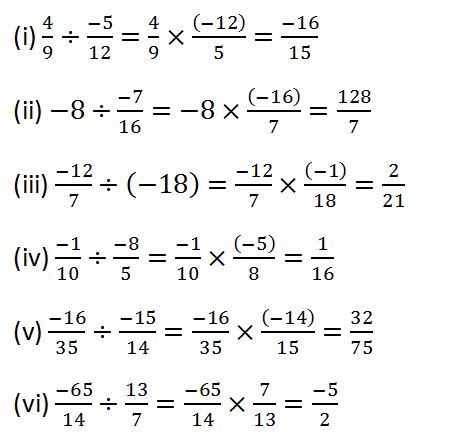# RS Aggarwal Class 8 Math First Chapter Rational Numbers Exercise 1E Solution

## EXERCISE 1E

(1) Simplify:(2) Verify whether the given statement is true or false:(3) Verify whether the given statement is true or false:(4) The product of two rational numbers is – 9. If one of the numbers is – 12, find the other.(5) The product of two rational numbers is (-16)/9. If one of the numbers is (-4)/3, find the other.(6) By what rational number should we multiply (-15)/56 to get (-5)/7?(7) By what rational number should (-8)/39 be multiplied to obtain 1/26?(8) By what number should (-33)/8 be divided to get (-11)/2?(9) Divide the sum of 13/5 and (-12)/7 by the product of (-31)/7 and 1/(-2).(10) Divide the sum of 65/12 and 8/3 by their difference.(11) Fill in the blanks:(12) (i) Are rational numbers always closed under division?

Ans: No

(ii) Are rational numbers always commutative under division?

Ans: No

(iii) Are rational numbers always associative under division?

Ans: No

(iv) Can we divide 1 by 0?

Ans: No

Updated: May 30, 2022 — 1:54 pm